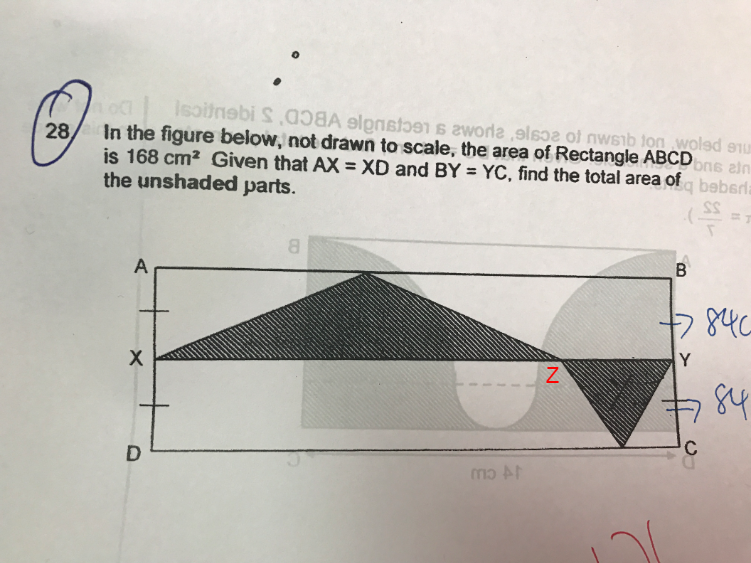# Question

Given that AX = XD and BY = YC, the shaded parts = 1/2 of ABYX = 1/4 of ABCD.
total area of the unshaded parts ——- (3/4) x 168 = 126

Ans : 126 sq cm.

Move the triangle from the bottom right to the ABYX, then draw a perpendicular line across the rectangle from the point where the two triangles meet to form two smaller rectangles. The area of each of the triangles will be half the area of the respective smaller rectangles. 🙂

0 Replies 0 LikesArea of big shaded triangle → ½(XZ)(AX)

Area of small shaded triangle → ½(ZY)(XD) = ½(ZY)(AX)   (since AX = XD)

Total shaded area → ½(XZ)(AX) + ½(ZY)(AX) = ½(AX)(XZ + ZY)

XZ + ZY = XY

Total shaded area → ½(AX)(XY) = ½ of ABYX = ¼ of ABCD

Unshaded area → ¾ of ABCD = ¾ × 168 = 126 cm2

0 Replies 0 Likes